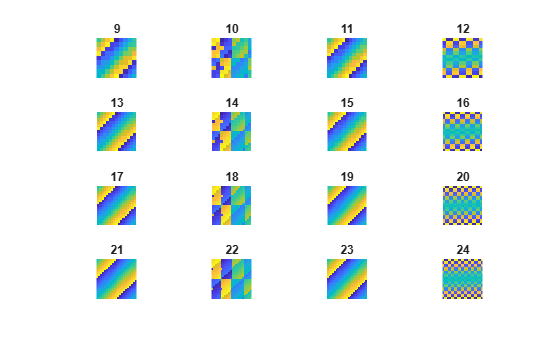# magic

## Syntax

``M = magic(n)``

## Description

example

````M = magic(n)` returns an `n`-by-`n` matrix constructed from the integers `1` through `n`2 with equal row and column sums. The order `n` must be a scalar greater than or equal to `3` in order to create a valid magic square.```

## Examples

collapse all

Compute the third-order magic square `M`.

`M = magic(3)`
```M = 3×3 8 1 6 3 5 7 4 9 2 ```

The sum of the elements in each column and the sum of the elements in each row are the same.

`sum(M)`
```ans = 1×3 15 15 15 ```
`sum(M,2)`
```ans = 3×1 15 15 15 ```

Visually examine the patterns in magic square matrices with orders between 9 and 24 using `imagesc`. The patterns show that `magic` uses three different algorithms, depending on whether the value of `mod(n,4)` is 0, 2, or odd.

```for n = 1:16 subplot(4,4,n) ord = n+8; m = magic(ord); imagesc(m) title(num2str(ord)) axis equal axis off end```## Input Arguments

collapse all

Matrix order, specified as a scalar integer greater than or equal to 3. If `n` is complex, not an integer, or not scalar, then `magic` converts it into a usable integer with `floor(real(double(n(1))))`.

If you supply `n` less than `3`, then `magic` returns either a nonmagic square, or the degenerate magic squares `1` and `[]`.

Data Types: `single` | `double` | `int8` | `int16` | `int32` | `int64` | `uint8` | `uint16` | `uint32` | `uint64` | `logical` | `char`

## Version History

Introduced before R2006a